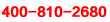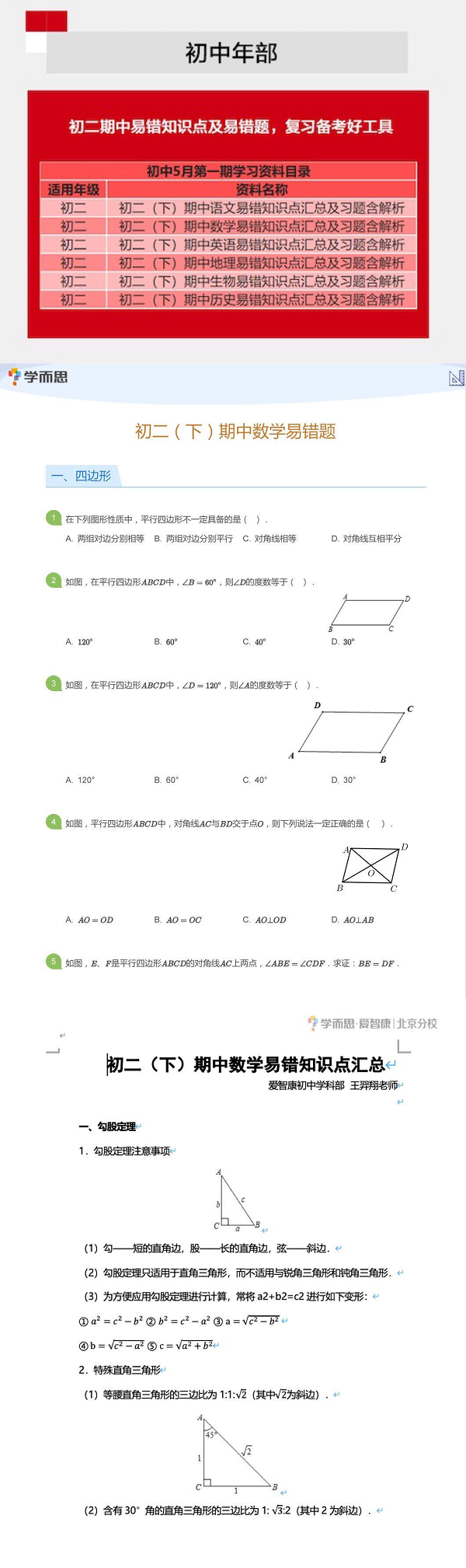# 北京初中三角函数教学视频

2020-05-04 14:06:17 　来源：百度文库点击领取→北京初二下期中复习资料合集

中考数学复习辅导：三角函数诱导公式

诱导公式的本质

所谓三角函数诱导公式，就是将角n·(π/2)±α的三角函数转化为角α的三角函数。

常用的诱导公式

公式一：设α为任意角，终边相同的角的同一三角函数的值相等：

sin(2kπ+α)=sinαk∈z

cos(2kπ+α)=cosαk∈z

tan(2kπ+α)=tanαk∈z

cot(2kπ+α)=cotαk∈z

公式二：设α为任意角，π+α的三角函数值与α的三角函数值之间的关系：

sin(π+α)=-sinα

cos(π+α)=-cosα

tan(π+α)=tanα

cot(π+α)=cotα

公式三：任意角α与-α的三角函数值之间的关系：

sin(-α)=-sinα

cos(-α)=cosα

tan(-α)=-tanα

cot(-α)=-cotα

公式四：利用公式二和公式三可以得到π-α与α的三角函数值之间的关系：

sin(π-α)=sinα

cos(π-α)=-cosα

tan(π-α)=-tanα

cot(π-α)=-cotα

公式五：利用公式一和公式三可以得到2π-α与α的三角函数值之间的关系：

sin(2π-α)=-sinα

cos(2π-α)=cosα

tan(2π-α)=-tanα

cot(2π-α)=-cotα

公式六：π/2±α与α的三角函数值之间的关系：

sin(π/2+α)=cosα

cos(π/2+α)=-sinα

tan(π/2+α)=-cotα

cot(π/2+α)=-tanα

sin(π/2-α)=cosα

cos(π/2-α)=sinα

tan(π/2-α)=cotα

cot(π/2-α)=tanα

推算公式：3π/2±α与α的三角函数值之间的关系：

sin(3π/2+α)=-cosα

cos(3π/2+α)=sinα

tan(3π/2+α)=-cotα

cot(3π/2+α)=-tanα

sin(3π/2-α)=-cosα

cos(3π/2-α)=-sinα

tan(3π/2-α)=cotα

cot(3π/2-α)=tanα

诱导公式记忆口诀：“奇变偶不变，符号看象限”。

“奇、偶”指的是π/2的倍数的奇偶，“变与不变”指的是三角函数的名称的变化：“变”是指正弦变余弦，正切变余切。(反之亦然成立)“符号看象限”的含义是：把角α看做锐角，不考虑α角所在象限，看n·(π/2)±α是第几象限角，从而得到等式右边是正号还是负号。

符号判断口诀：

“一全正;二正弦;三两切;四余弦”。这十二字口诀的意思就是说：第一象限内任何一个角的四种三角函数值都是“+”;第二象限内只有正弦是“+”，其余全部是“-”;第三象限内只有正切和余切是“+”，其余全部是“-”;第四象限内只有余弦是“+”，其余全部是“-”。

“ASCT”反Z。意即为“all(全部)”、“sin”、“cos”、“tan”按照将字母Z反过来写所占的象限对应的三角函数为正值。

三角函数重要知识点总结

1、勾股定理：直角三角形两直角边a、b的平方和等于斜边c的平方a2+b2=c2。

2、如下图，在Rt△ABC中，∠C为直角，则∠A的锐角三角函数为(∠A可换成∠B)：

3、任意锐角的正弦值等于它的余角的余弦值;任意锐角的余弦值等于它的余角的正弦值。

4、任意锐角的正切值等于它的余角的余切值;任意锐角的余切值等于它的余角的正切值。

5、0°、30°、45°、60°、90°特殊角的三角函数值(重要)

6、正弦、余弦的增减性：

当0°≤α≤90°时，sinα随α的增大而增大，cosα随α的增大而减小。

7、正切、余切的增减性：当0°<α<90°时，tanα随α的增大而增大，cotα随α的增大而减小。

同学们距离中考越来越近了，同学们对初中三年所学的知识复习的怎么样了？想了解相关课程的同学，请拨打学而思爱智康免费咨询电话：点击领取：《北京初二下期中复习资料合集》复习资料QQ扫一扫您将获得群内福利家长互动海量资料同步免费视频课程了解最新信息及时解读资讯你可能感兴趣的文章立即咨询1对1在线课程*学而思爱智康会在24小时内与您取得电话联系大家都在看热门课程介绍限时免费领取学习相关

• 全国课程在线咨询
• 咨询热线：4000-121-121
• 扫描注册有礼

• 让学习更有效